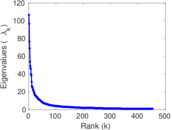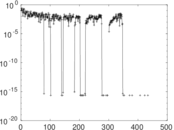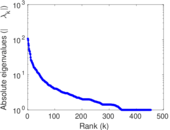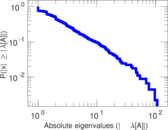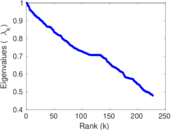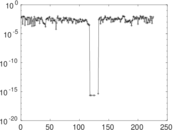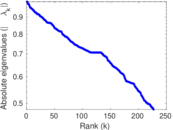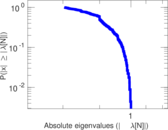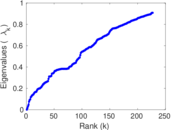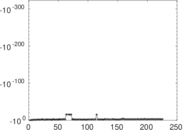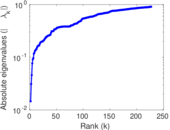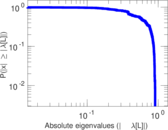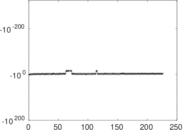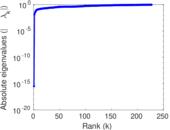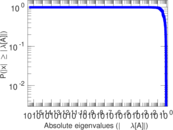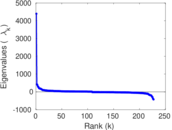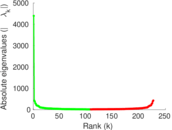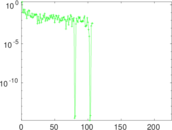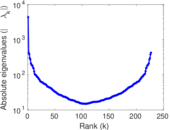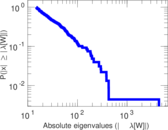# Wikiquote edits (id)

This is the bipartite edit network of the Indonesian Wikiquote. It contains users and pages from the Indonesian Wikiquote, connected by edit events. Each edge represents an edit. The dataset includes the timestamp of each edit.

 Code `qid` Internal name `edit-idwikiquote` Name Wikiquote edits (id) Data source http://dumps.wikimedia.org/ AvailabilityDataset is available for download Consistency checkDataset passed all tests Category Authorship network Dataset timestamp 2017-10-20 Node meaning User, article Edge meaning Edit Network formatBipartite, undirected Edge typeUnweighted, multiple edges Temporal dataEdges are annotated with timestamps

## Statistics

 Size n = 4,414 Left size n1 = 683 Right size n2 = 3,731 Volume m = 11,668 Unique edge count m̿ = 7,625 Wedge count s = 809,549 Claw count z = 96,288,983 Cross count x = 10,362,837,951 Square count q = 184,896 4-Tour count T4 = 4,736,398 Maximum degree dmax = 941 Maximum left degree d1max = 941 Maximum right degree d2max = 165 Average degree d = 5.286 81 Average left degree d1 = 17.083 5 Average right degree d2 = 3.127 31 Fill p = 0.002 992 22 Average edge multiplicity m̃ = 1.530 23 Size of LCC N = 3,784 Diameter δ = 12 50-Percentile effective diameter δ0.5 = 3.697 03 90-Percentile effective diameter δ0.9 = 5.644 67 Median distance δM = 4 Mean distance δm = 4.381 62 Gini coefficient G = 0.721 250 Balanced inequality ratio P = 0.216 790 Left balanced inequality ratio P1 = 0.120 758 Right balanced inequality ratio P2 = 0.302 451 Relative edge distribution entropy Her = 0.818 235 Power law exponent γ = 2.854 35 Tail power law exponent γt = 2.291 00 Tail power law exponent with p γ3 = 2.291 00 p-value p = 0.000 00 Left tail power law exponent with p γ3,1 = 1.831 00 Left p-value p1 = 0.002 000 00 Right tail power law exponent with p γ3,2 = 2.791 00 Right p-value p2 = 0.000 00 Degree assortativity ρ = −0.224 073 Degree assortativity p-value pρ = 2.182 45 × 10−87 Spectral norm α = 106.595 Algebraic connectivity a = 0.014 562 3 Spectral separation |λ1[A] / λ2[A]| = 1.103 76 Controllability C = 3,105 Relative controllability Cr = 0.728 360

## Plots

### Fruchterman–Reingold graph drawing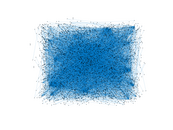### Degree distribution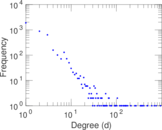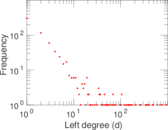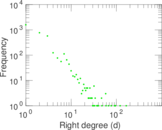### Cumulative degree distribution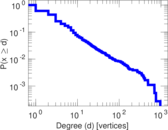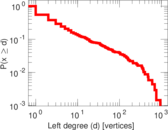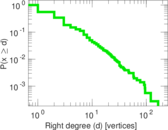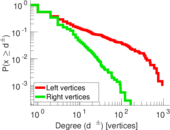### Lorenz curve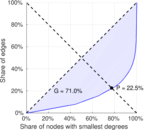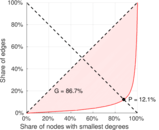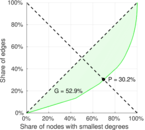### Spectral distribution of the adjacency matrix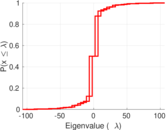### Spectral distribution of the normalized adjacency matrix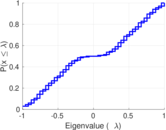### Spectral distribution of the Laplacian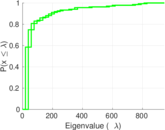### Spectral graph drawing based on the adjacency matrix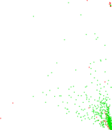### Spectral graph drawing based on the Laplacian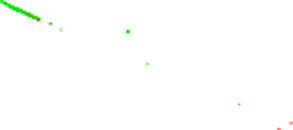### Spectral graph drawing based on the normalized adjacency matrix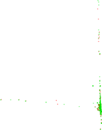### Degree assortativity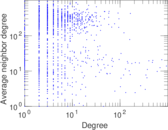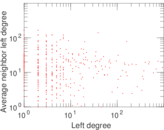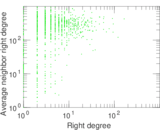### Zipf plot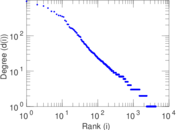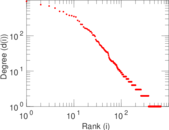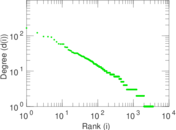### Hop distribution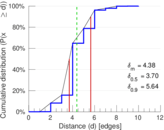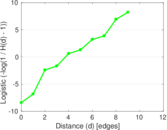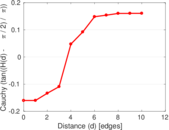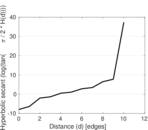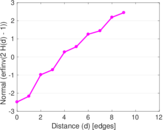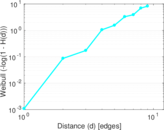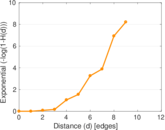### Double Laplacian graph drawing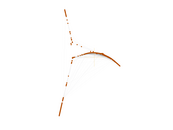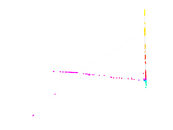### Delaunay graph drawing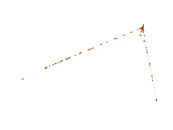### Edge weight/multiplicity distribution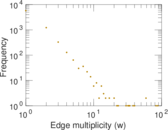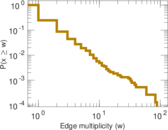### Temporal distribution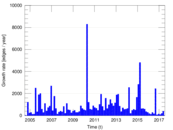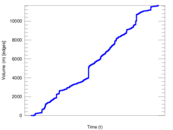### Temporal hop distribution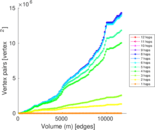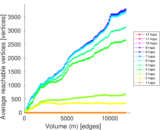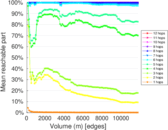### Diameter/density evolution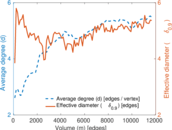### Matrix decompositions plots